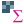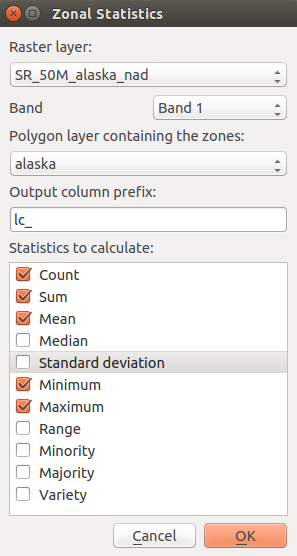` `

# Zonal Statistics Plugin¶

With theZonal statistics plugin, you can analyze the results of a thematic classification. It allows you to calculate several values of the pixels of a raster layer with the help of a polygonal vector layer (see figure_zonal_statistics). Choosing a color band, the plugin generates output columns in the vector layer with an user-defined prefix and calculates for each polygon, statistics on pixels that are within. The available statistics are :

• Count: to count the number of pixels
• Sum: to sum the pixel values
• Mean: to get the mean of pixel values
• Median: to get the median of pixel values
• StDev: to get the standard deviation of pixel values
• Min: to get the minimum of pixel values
• Max: to get the maximum of pixel values
• Range: to get the range (max - min) of pixel values
• Minority: to get the less represented pixel value
• Majority: to get the most represented pixel value
• Variety: to count the number of distinct pixel valuesZonal statistics dialog